• 目录1 概述2 例题2.1 有效的字母异位词2.2 数字之和欢迎使用Markdown编辑器新的改变功能快捷键合理的创建标题，有助于目录的生成如何改变文本的样式插入链接与图片如何插入一段漂亮的代码片生成一个适合你的列表创建...


目录
1 概述1.1 HashMap实现方式1.2 Hash碰撞1.3 更多实现方式
2 例题2.1 有效的字母异位词2.2 数字之和2.3 K数之和

1 概述
该博客结合leetcode原题介绍了如何利用哈希表降低程序的时间复杂度。
1.1 HashMap实现方式
将key通过一个function：变成一个数字（429），存入大小为30的数组中（取余数得到数组中的下标）。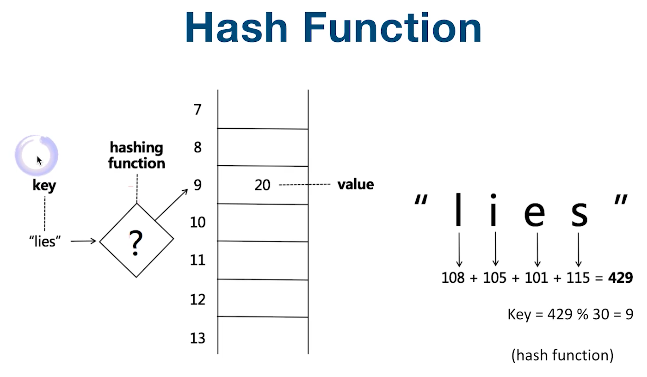1.2 Hash碰撞
Function的设计有可能算出重复的数组下标（尽量避免），有许多方法可以解决。此处介绍“拉链法”，即在同一个数组下标处存一个链表，将重复的key链起来。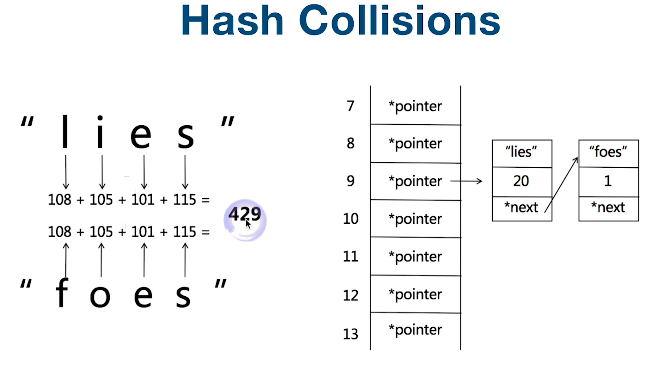1.3 更多实现方式
set和map类似，可以用Hash和Tree两种方式实现。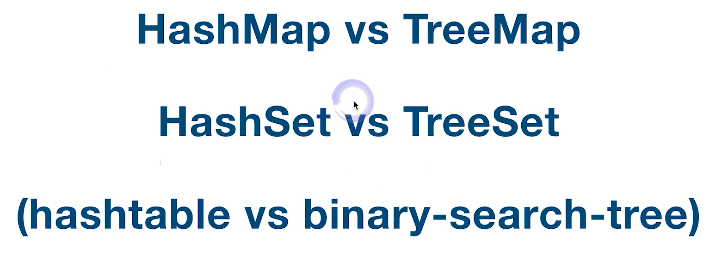Hash的时间复杂度O（1），Tree的时间复杂度O（log（N）），但是Tree是有序排列，Hash是无序排列。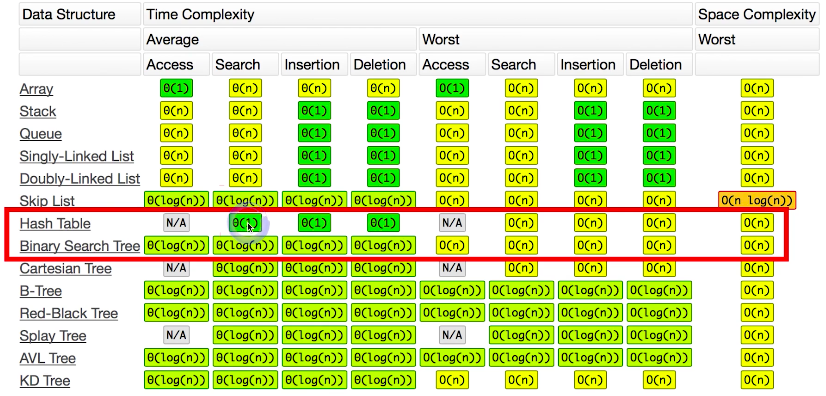2 例题
2.1 有效的字母异位词
#Leetcode 242 有效的字母异位词 该题是让我们判断两个字符串是否可以通过字母顺序变换得到一样的结果。 （1）暴力解法 两个字符串分别排序，然后看是否相等。 *时间复杂度：O(NlogN) *空间复杂度：O（1）
# coding = utf-8
class Solution(object):
def isAnagram(self, s, t):
"""
:type s: str
:type t: str
:rtype: bool
"""
return sorted(s)==sorted(t)

（2）利用hashmap 分别遍历两个字符串，将对应字母以及出现的总次数记录在字典中，然后比较字典是否相同。 *时间复杂度：O（N） *空间复杂度：O（N）
# coding = utf-8
class Solution(object):
def isAnagram(self, s, t):
"""
:type s: str
:type t: str
:rtype: bool
"""
if len(s)!=len(t):
return False
s_map, t_map = {}, {}
for c in s:
if c in s_map:
s_map[c] += 1
else:
s_map[c] = 1
for c in t:
if c in t_map:
t_map[c] += 1
else:
t_map[c] = 1
if s_map==t_map:
return True
else:
return False

2.2 数字之和
#Leetcode 1，15 两数之和，三数之和（高频题） 该题是让我们判断给定的数组中是否可以找到数字的组合满足求和等于target（该题target是0），并返回所有不重复的组合，下面我们以三数之和为例展开。 （1）暴力解法 对数组做三层循环，i从0开始，j从i+1开始，k从j+1开始，遍历完，找到所有的组合，最后去重。（此处使用了一个小技巧，先将数组排序，用set存储结果，避免最后去重的时间复杂度过大） *时间复杂度：O（N^3） *空间复杂度：O（1）
def threeSum(self, nums):
if len(nums)<3:
return []
nums.sort()
res = set()
for i in range(0, len(nums)):
for j in range(i+1, len(nums)):
for k in range(j+1, len(nums)):
if nums[i]+nums[j]+nums[k]==0:
return map(list, res)

（2）使用hashmap 该解法考虑到遍历第1,2层时用target减去这两个数即所找的数，因此使用hashmap存储数组可以节省一层循环 *时间复杂度：O（N^2） *空间复杂度：O（N）
def threeSum(self, nums):
if len(nums)<3:
return []
d = {}
res = set()
for i, v in enumerate(nums):
d[v] = 1
for i in range(0, len(nums)):
for j in range(i+1, len(nums)):
if -nums[i]-nums[j] in d:
return map(list, res)

（3）两边往中间夹 比较反人类的做法，首先排序，依次遍历每个元素，此次观察后续数组的首尾，大于0则尾元素减一位，小于0则首元素加一位。 *时间复杂度：O（N^2） *空间复杂度：O（1）
def threeSum(self, nums):
if len(nums) < 3:
return []
nums.sort()
res = set()
for i, v in enumerate(nums):
if i>=len(nums)-1: break
j = i + 1
k = len(nums) - 1
while j < k:
if nums[i] + nums[j] + nums[k] > 0:
k -= 1
elif nums[i] + nums[j] + nums[k] < 0:
j += 1
else:
j += 1
k -= 1
return map(list, res)

2.3 K数之和
该题是面试中经常遇到，不在leetcode中出现，原则是将此问题抽象为递归，最小问题就是2-sum。

public void kSum(int[] nums, int k, int target, int from, int end, List<Integer> cur, List<List<Integer>> result){
if(k == 2) {
int left = from;
int right = end;
while(left < right){
int diff = target- nums[left] - nums[right];
if (diff == 0){
List<Integer> r = new ArrayList<>();
while(left < right && nums[left] == nums[left + 1]) left++;
while(left < right && nums[right] == nums[right - 1]) right--;
left++;
right--;
}else if (diff > 0){
left++;
}else {
right--;
}
}
}else {  // k>2时
for(int i = from; i < end - k + 2; i++){
if(i > from && nums[i] == nums[i - 1]) continue;
kSum(nums, k - 1, target - nums[i], i + 1, end, cur, result);
cur.remove(cur.size() - 1);
}
}
}

展开全文• 哈希表存储的基本思想是：以数据表中的每个记录的关键字 k为自变量，通过一种函数H(k)计算出函数值。把这个值解释为一块连续存储空间（即数组空间）的单元地址（即下标），将该记录存储到这个单元中。在此称该函数H....

哈希表类概念摘要
哈希表类SqHash的建立、查找。设有若干个学生的考试成绩，采用除留余数求哈希地址，将学生的信息存储到该地址空间，并且采用线性探测法解决冲突问题。
哈希表又称散列表。
哈希表存储的基本思想是：以数据表中的每个记录的关键字 k为自变量，通过一种函数H(k)计算出函数值。把这个值解释为一块连续存储空间（即数组空间）的单元地址（即下标），将该记录存储到这个单元中。在此称该函数H为哈希函数或散列函数。按这种方法建立的表称为哈希表或散列表。
除留余数法:
取关键字k被某个不大于表长m的数p除后所得余数作为哈希函数地址的方法。即：
H（k）＝k  mod ｐ
这种方法的关键是选择好p。使得数据集合中的每一个关键字通过该函数转化后映射到哈希表的任意地址上的概率相等。理论研究表明，一般取p为小于ｍ的最大质数或不包含小于20的质因素的合数。
线性探测再散列:
当发生冲突时，将依次探测“下一个位置”，直到找到其关键字相匹配的元素或找到一个空位插入。设哈希空间长度为m，“下一个位置”由下式确定：
Hi=(H(key)+di) mod m
H(key):哈希函数
m:哈希表长度
di：求“下一个位置”的增量
di=1，2，…，m-1。
这种di的取法称为线性探测再散列。即“下一个位置”为哈希表的直接后继。若当di=m-1时仍未查到，则说明表满，还要查找另外的溢出表。缺点：容易产生“二次聚集”

我来简单总结一下这个究竟讲了什么这次的内容是查找和排序那么跟查找有关的有哈希，跟排序有关的有冒泡排序，快速排序关于哈希的工作原理，大家可以仔细阅读上面那段话那么下面先贴出的是
哈希查找的演示代码
#include <iostream.h>
#include <conio.h>
#include <string.h>
#include <iomanip.h>
//---------------------------------------------------------------------------
typedef int KeyType;       // 关键字的类型
const int MAXSIZE=100;     // 数组的容量
struct ElemType            //学生的记录信息
{ KeyType  key ;        //学号
char name;         //姓名
int english;          //成绩
int math;             //成绩
} ;
class SqHash
{ private:
ElemType *ht;     // 哈希表数组
int length;       // 哈希表容量
KeyType   p;      // 除留余数法的大质数
public:
SqHash( int n1,int p1);
~SqHash(){delete []ht;  length=0;};
int  find( KeyType K );
void  creat_hash();
void PrintOut();
};
//-------------------------------------------------------------
SqHash::SqHash(int n1,int p1)
{ length=n1;  p=p1;
ht=new ElemType[length];
for(int i=0;i<length;i++)ht[i].key=0;
}
void  SqHash::creat_hash()
{ int i,j,K,en,ma;char na;
cout<<"\n  请逐一输入各个学号（关键字值）（-1结束）："; cin>>K;
while(K!=-1)
{  i=find(K);
if(i==-32767)  cout<<"\n   成功查找到,不再插入！";
else  if(i!=32767){
cout<<"\n  请输入学生的姓名，英语成绩和高数成绩：";    cin>>na>>en>>ma;
ht[i].key=K;
strcpy(ht[i].name,na);     //用串拷贝赋值
ht[i].english=en;
ht[i].math=ma;              //　插入学生记录K
cout<<"\n   插入成功！" ;
cout<<"\n  请逐一输入各个学号（关键字值）（-1结束）："; cin>>K;
}
}
}
int  SqHash::find( KeyType K )
{
int i,j;
j=K%p;
i=j-1;
while((ht[j].key!=0)&&(ht[j].key!=K)&&(j!=i))
j=(j+1)%MAXSIZE;//解决冲突
if(ht[j].key==K)
{
cout<<"\n  成功查找到学号为"<<K<<"的学生，位置在："<<j<<"上";
cout<<"\n\n 该学生的基本信息为：\n\n";
cout<<"姓名："<<ht[j].name<<"英语成绩:"<<ht[j].english<<"高数成绩："<<ht[j].math;
j=-32767;
}
else if(j==i){
cout<<"\n 表满，出现异常！ OverFlow!";//溢出
j=32767;
}
return j;

}
void  SqHash::PrintOut()
{ int i,j;
for (i=0;i<length; i++)
cout<<"\n  i="<<i<<"   学号："<<setw(6)<<ht[i].key<<"   姓"<<setw(6)<<ht[i].name
<<"   英语成绩："<<setw(6)<<ht[i].english<<"   高数成绩："<<setw(6)<<ht[i].math;
}
int main()
{  int p0,n0;
cout<<"\n  请输入n值（n值应是记录总数的1.3-1.5倍)"; cin>>n0;
cout<<"\n  请输入P值（应是不大于n 的大质数）："; cin>>p0;
SqHash  ha(n0,p0);  ElemType a;
int k; char ch;
do { cout<<"\n\n\n";
cout<<"\n       1. 建立哈希表（开放地址法）  ";
cout<<"\n       2. 在哈希表中查找某位学生 ";
cout<<"\n       3. 输出哈希表";
cout<<"\n       4. 结束";
cout<<"\n=======================================";
cout<<"\n       输入您的选择(1,2,3,4):"; cin>>k;
switch(k)
{ case 1:{  ha.creat_hash();
}  break;
case 2:{  cout<<"\n  请输入待查找的学生学号：";  cin>>a.key;
int i=ha.find(a.key);
if(i!=-32767) cout<<"\n  此学生"<<a.key<<"  不存在！" ;
}   break;
case 3:{ ha.PrintOut(); }  break;
}
}while(k>=1&&k<=3);
_getch();        return 0;
}

冒泡排序（Bubble Sort），是一种计算机科学领域的较简单的排序算法。
它重复地走访过要排序的元素列，依次比较两个相邻的元素，如果他们的顺序（如从大到小、首字母从A到Z）错误就把他们交换过来。走访元素的工作是重复地进行直到没有相邻元素需要交换，也就是说该元素已经排序完成。
这个算法的名字由来是因为越大的元素会经由交换慢慢“浮”到数列的顶端（升序或降序排列），就如同碳酸饮料中二氧化碳的气泡最终会上浮到顶端一样，故名“冒泡排序”。

冒泡法排序演示例子
#include<iostream.h>
class intsz
{
public:
int i;
};

int main()
{
int j,t;
intsz i;
cout<<"请输入你需要输入的数据个数总数：";
cin>>j;
for(int a=0;a<j;a++)
{
cout<<"请输入第"<<a+1<<"个数值：";
cin>>i[a];
}
for(int b=0;b<j;b++)
for(int c=0;c<j;c++)
{
if(i[b]<i[c])
{
t=i[b];
i[b]=i[c];
i[c]=t;
}
}

cout<<"从小到大排序:"<<endl;
for(a=0;a<j;a++)
{
cout<<i[a]<<"  ";
}

cout<<endl<<"从大到小排序:"<<endl;
j--;
for(a=0;a<j+1;j--)
{
cout<<i[j]<<"  ";
}
cout<<endl;

return 0;

}

排序除了冒泡排序外还有快速排序、希尔排序

快速排序概念
快速排序由C. A. R. Hoare在1962年提出。它的基本思想是：通过一趟排序将要排序的数据分割成独立的两部分，其中一部分的所有数据都比另外一部分的所有数据都要小，然后再按此方法对这两部分数据分别进行快速排序，整个排序过程可以递归进行，以此达到整个数据变成有序序列。
设要排序的数组是A……A[N-1]，首先任意选取一个数据（通常选用数组的第一个数）作为关键数据，然后将所有比它小的数都放到它前面，所有比它大的数都放到它后面，这个过程称为一趟快速排序。值得注意的是，快速排序不是一种稳定的排序算法，也就是说，多个相同的值的相对位置也许会在算法结束时产生变动。
一趟快速排序的算法是：
1）设置两个变量i、j，排序开始的时候：i=0，j=N-1；
2）以第一个数组元素作为关键数据，赋值给key，即key=A；
3）从j开始向前搜索，即由后开始向前搜索(j--)，找到第一个小于key的值A[j]，将A[j]和A[i]互换；
4）从i开始向后搜索，即由前开始向后搜索(i++)，找到第一个大于key的A[i]，将A[i]和A[j]互换；
5）重复第3、4步，直到i=j； (3,4步中，没找到符合条件的值，即3中A[j]不小于key,4中A[i]不大于key的时候改变j、i的值，使得j=j-1，i=i+1，直至找到为止。找到符合条件的值，进行交换的时候i， j指针位置不变。另外，i==j这一过程一定正好是i+或j-完成的时候，此时令循环结束）。

快速排序又分为两种，一种是递归，一种是非递归 1.递归快速排序算法：略，如果需要的朋友可以留言（或者自己去百度） 2.非递归快速排序算法：
#include <iostream.h>
#include  <conio.h>
struct node  //记录结构。为简化问题，设定记录中只含关键字
{int key;
}a;       //a数组是全局变量
int n;            //n也是全局变量
void print( )
{int i;
for (i=0;i<n;i++) cout<<a[i].key<<" ";
cout<<"\n";
}
void creat()
{int i;   n=0;
cout<<"input keys"; cin>>i;
while (i!=-9999)  {a[n].key=i; n++;
cin>>i;
}
}
template <class T>
int hoare(T a,int l,int h) /*分区处理函数*/
{int i,j;    node x;
i=l;j=h;x.key=a[i].key;
do{   while((i<j) && (a[j].key>=x.key)) j--;
if(i<j) {a[i].key=a[j].key;i++;}
while((i<j) && (a[i].key<=x.key)) i++;
if (i<j) {a[j].key=a[i].key;j--;}
}while (i<j);
a[i].key=x.key;  return i;
}//hoare end
template <class T>
void quick1(T a,int n)  //非递归的快速排序主体函数
{int i,l,h,tag,top;
int s;
l=0;h=n-1;tag=1;top=0;
do {while (l<h)  {i=hoare(a,l,h);
top++;  s[top]=i+1;  s[top]=h;h=h-1;
}
if(top==0) tag=0;
else { l=s[top];
h=s[top];  top--;
}
}while (tag==1);
cout<<"\n  输出非递归快速排序的结果:"<<endl;
}//quick1 end
int  main()
{  creat();  print();
quick1(a,n); print();     // a数组和n都是全局变量
_getch();   return 0;
}

希尔排序
希尔排序(Shell's Sort)是插入排序的一种又称“缩小增量排序”（Diminishing Increment Sort），是直接插入排序算法的一种更高效的改进版本。希尔排序是非稳定排序算法。该方法因D.L.Shell于1959年提出而得名。
希尔排序是把记录按下标的一定增量分组，对每组使用直接插入排序算法排序；随着增量逐渐减少，每组包含的关键词越来越多，当增量减至1时，整个文件恰被分成一组，算法便终止。
先取一个小于n的整数d1作为第一个增量，把文件的全部记录分组。所有距离为d1的倍数的记录放在同一个组中。先在各组内进行直接插入排序；然后，取第二个增量d2<d1重复上述的分组和排序，直至所取的增量=1(<…<d2<d1)，即所有记录放在同一组中进行直接插入排序为止。
该方法实质上是一种分组插入方法
比较相隔较远距离（称为增量）的数，使得数移动时能跨过多个元素，则进行一次比较就可能消除多个元素交换。D.L.shell于1959年在以他名字命名的排序算法中实现了这一思想。算法先将要排序的一组数按某个增量d分成若干组，每组中记录的下标相差d.对每组中全部元素进行排序，然后再用一个较小的增量对它进行，在每组中再进行排序。当增量减到1时，整个要排序的数被分成一组，排序完成。
一般的初次取序列的一半为增量，以后每次减半，直到增量为1。
给定实例的shell排序的排序过程
假设待排序文件有10个记录，其关键字分别是：
49，38，65，97，76，13，27，49，55，04。
增量序列的取值依次为：5，2，1

希尔排序演示代码：
#include <iostream.h>
#include  <conio.h>
struct node  //记录结构。为简化问题，设定记录中只含关键字
{int key;
}a;
int n;
void print( )
{ int i;
for (i=0;i<n;i++)  cout<<a[i].key<<endl
}
void creat()
{int i; n=0;
cout<<"input keys:"; cin>>i;
while (i!=-9999)  {a[n].key=i;n++;
cin>>i;
}
}
template <class T>
void shell(T a[n],int n)  //希尔排序
{ int i,j,k;
for(i=n;i>=1;i--) a[i].key=a[i-1].key;
k=n/2;
while (k>=1)
{ for (i=k+1;i<=n;i++)
{ a.key=a[i].key; j=i-k;
while((a[j].key>a.key)&&(j>=0))
{ a[j+k].key=a[j].key; j=j-k; }
a[j+k]=a;
}
k=k/2;
}
for (i=0;i<n;i++) a[i].key=a[i+1].key;
cout<<"\n  输出希尔排序的结果:"<<endl;
}//shell end
int  main()
{  creat();  print();
shell(a,n); print();
_getch();  return 0;
}

到这里我们已经基本上已经将常见的和常用的查找算法以及排序算法讲解完了，对于一般人来说，基本掌握这几种算法就可以了。
关于如何去理解哈希算法的思想，这个需要慢慢的理解，初次接触这类算法或多或少都会有很多问题，或者看不懂，不理解的地方。
展开全文查找 排序 冒泡排序 快速排序
• 用unordered_map容器来使用哈希


目录标题
unordered_map关联式容器1. 文档介绍2. 接口说明2.1 构造2.2 容量2.3 迭代器2.4 元素访问2.5 查询2.6 修改操作2.7 桶操作
3. 底层结构4. 哈希表代码实现1. 开放定址法中的删除2. 线性探测的实现3. 链地址法4. 开散列与闭散列的比较5. 存储其他类型
参考文章

unordered_map关联式容器
1. 文档介绍
unorder_map是存储<key, value>键值对的关联式容器，其允许通过key快速的索引到与其对应的value键和映射值的类型可能不同，键值通常用于唯一的标识元素，而映射值是一个对象在内部unorder_map没有对<key, value>按照任何特定的顺序排序,为了在常数范围内找到key所对应的value，unorder_map将相同哈希值的键值对放在相同的桶中unorder_map容器通过key访问单个元素要比map快，但它通常在遍历元素子集的范围迭代方面效率较低unorder_map实现了直接访问操作符(operator[])，它允许使用key作为参数直接访问value，map也可以它的迭代器至少是前向迭代器hash的性能非常出色:拥有高达O(1)的插入和查找复杂度.这比map(平衡二叉树)的O(logn)要快
2. 接口说明
2.1 构造
#include<unordered_map>
unordered_map<T1, T2> mp;

2.2 容量
函数声明功能介绍bool empty() const检测容器是否为空size_t size() const获取容器中的有效元素个数
2.3 迭代器
函数声明功能介绍begin返回第一个元素的迭代器end返回最后一个元素下一个位置的迭代器cbegin返回第一个元素的const迭代器cend返回最后一个元素下一个位置的const迭代器
2.4 元素访问
函数声明功能介绍operator[]返回与key对应的value，没有一个默认值
2.5 查询
函数声明功能介绍iterator find(const K& key)返回key在哈希桶中的位置size_t count(const K& key)返回哈希桶中关键码为key的键值对的个数，查看是否存在

unordered_map中key是不能重复的，因此count函数的返回值最大为1

2.6 修改操作
函数声明功能介绍insert向容器中插入键值对erase删除容器中的键值对void clear()清空容器中有效元素个数void swap(unorder_map&)交换两个容器中的元素
2.7 桶操作
函数声明功能介绍size_t bucket_count() const返回哈希桶中桶的总个数size_t bucket_size(size_t n) const返回n号桶中有效元素的总个数size_t bucket(const K& key)返回元素key所在的桶号
3. 底层结构
unorder系列的关联式容器之所以效率比较高，是因为其底层使用了哈希结构 map/multimap属于关联式容器，底层结构是用二叉树实现
4. 哈希表代码实现
1. 开放定址法中的删除
// 懒惰删除，增加一个删除标记
// 哈希表每个空间给个标记
// EMPTY此位置为空，EXIST此位置已经有元素，DELETE元素已经删除
enum Status{EMPTY, EXIST, DELETE};

2. 线性探测的实现
#include<iostream>
#include<vector>
using namespace std;

// hash设计时要尽可能少冲突，非素数有一对公约数，冲突概率暴涨，因此capacity要是素数(质数)
// 素数列表，num_prime是个数
static const int num_prime = 32;
static const unsigned long prime_list[num_prime] =
{
3, 7, 13, 19,
53,         97,           193,         389,       769,
1543,       3079,         6151,        12289,     24593,
49157,      98317,        196613,      393241,    786433,
1572869,    3145739,      6291469,     12582917,  25165843,
50331653,   100663319,    201326611,   402653189, 805306457,
1610612741, 3221225473ul, 4294967291ul
};

// 根据输入的数得到比它大的素数
unsigned long GetNextPrime(size_t num)
{
for(int i=0; i<num_prime; i++)
{
if(prime_list[i] > num)
{
return prime_list[i];     // 返回第一个比num大的数
}
}
// 如果没有比num大的素数, 返回列表中最后一个数
return prime_list[num_prime - 1];
};

// 闭散列处理哈希冲突，不能随便物理删除哈希表中已有的元素，若直接删除元素会影响其他元素的搜索
// 所以每个空间要给个标记
enum Status
{
EMPTY,  // 空
EXIST,  // 有元素
DELETE  // 已经删除
};

template<class key, class value>
class HashTable{
struct Elem
{
pair<key, value> _val;         // 键值对
Status _status;
};
public:
// 形参设置默认值，函数调用时，不传递任何实参就使用默认参数，有实参则进行实参传递
// 初始化列表中，(number)是为容器开辟了number大小的空间
HashTable(size_t capacity = 3) : _ht(capacity), _size(0)
{
for(size_t i= 0; i<capacity; ++i)
_ht[i]._status = EMPTY;
}

public:
// 插入方法
// 闭散列，线性探测法
bool Insert(const pair<key, value>& val)
{
// 检测哈希表底层空间是否充足
CheckCapacity();
// 找到空的位置才可以插入
{
// 重复键值的元素是无法插入的
return false;

// 删除标记的地方可以重用
break;
/* 转一圈也没有找到，注意：动态哈希表，该种情况可以不用考虑，哈希表中元素个数到达
*一定的数量，哈希冲突概率会增大，需要扩容来降低哈希冲突，因此哈希表中元素是不会存满的
*/
}
// 插入元素
_size++;
return true;
}

// 获取size
size_t Size() const
{
return _size;
}
// 判断是否为空
bool Empty() const
{
return _size == 0;
}
// 查找
int Find(const key& t)
{
{
}
return -1;
}
// 删除
bool Erase(const key& k)
{
int index = Find(k);
if(index == -1)
return false;
_ht[index]._status = DELETE;
_size--;
return true;
}
// 交换
void Swap(HashTable<key, value>& ht)
{
swap(_ht, ht._ht);
swap(_size, ht._size);
}
private:
// 检查容量，不够就扩容
void CheckCapacity()
{
if(_size * 10 / _ht.capacity()  >= 7)
{
HashTable<key, value> newHt(GetNextPrime(_ht.capacity()));
for(size_t i=0; i<_ht.capacity(); i++)
{
if(_ht[i]._status == EXIST)
newHt.Insert(_ht[i]._val);
}
// 因为CheckCapacity()函数中的newHt对象是局部的，作用域只在这个函数中
// 局部变量存在栈区，编译器 编译结束后会释放掉
// 所以要将原来的对象和newHt对象进行交换
Swap(newHt);
}
}
// 哈希函数
size_t  HashFunc(const key& k)
{
return k % _ht.capacity();
}
private:
vector<Elem> _ht;
size_t _size;
};

int main()
{
cout << "111" << endl;
HashTable<int, int> ht;
int ar[] = { 4, 6, 8, 3, 6, 13, 1, 2, 9};
int n = sizeof(ar) / sizeof(int);
cout << n << endl;      // 9
for(int i=0; i<n; i++)
{
cout << "222" << endl;
ht.Insert(pair<int, int>(ar[i], ar[i]));
cout << "333" << endl;
}
// 因为不能有重复的元素,所以下面的结果是 8
cout << ht.Size() << endl;  //当前元素个数
ht.Erase(8);
cout << ht.Size() << endl;  //删除之后元素个数

cout <<"key = 6 : index = "<< ht.Find(6) << endl;
cout << "key = 8 : index = " << ht.Find(8) << endl;
cout <<"key = 13 : index = " << ht.Find(13) << endl;

return 0;
}

/*
unordered系列关联式容器的底层实现是hashtable，
比如在undered_set中value就是key，而在unordered_map中value代表键值对的值
哈希函数使用除留余数法计算存放地址，那么key就必须是整形或者转换为整形才能取模==
*/

3. 链地址法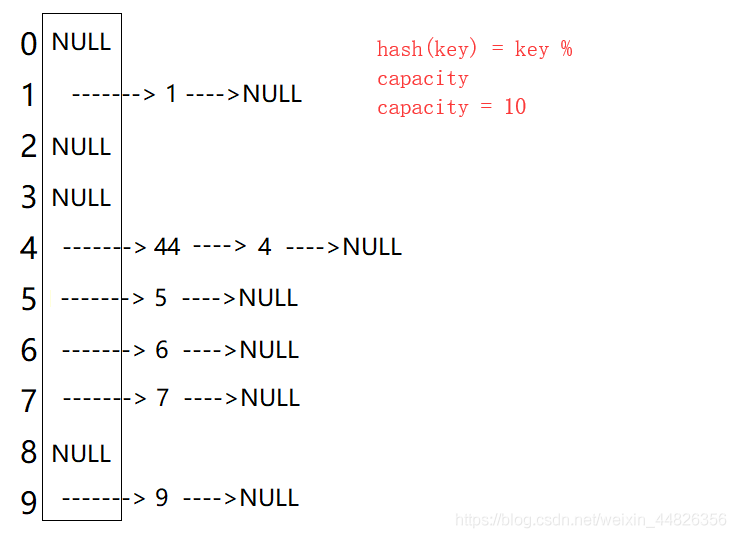#include<iostream>
#include<algorithm>
#include<vector>
#include<string>
using namespace std;

// 素数
static const int num_prime = 28;
static const unsigned long prime_list[num_prime] =
{
53,         97,           193,         389,       769,
1543,       3079,         6151,        12289,     24593,
49157,      98317,        196613,      393241,    786433,
1572869,    3145739,      6291469,     12582917,  25165843,
50331653,   100663319,    201326611,   402653189, 805306457,
1610612741, 3221225473ul, 4294967291ul
};

// 扩容的长度
inline unsigned long next_prime(unsigned long n)
{
const unsigned long* first = prime_list;
const unsigned long* last = prime_list + num_prime;
// lower_bound()函数的功能：
// 在[first, last)区间上返回一个存放不小于n的数的内存地址
const unsigned long* pos = lower_bound(first, last, n);
// 没找到返回最大值
return pos == last ? *(last-1) : *pos;
}

// 节点结构
template<class key_type, class value_type>
struct HashBucketNode
{
HashBucketNode<key_type, value_type>* _pNext;   // 指向下个节点的指针
pair<key_type, value_type> _val;        // 键值对
};

template<class key_type, class value_type>
class HashBucket
{
typedef struct HashBucketNode<key_type, value_type> Node;
public:
// 初始化
HashBucket(size_t capacity = 3) : _size(0)
{
const size_t n_buckets = next_prime(capacity);
// 预留空间
_ht.reserve(n_buckets);
// 插入节点结构的空指针
// insert(const_iterator pos, int count, ele);  // 迭代器指向位置pos插入count个元素ele
_ht.insert(_ht.end(), n_buckets, (Node*)0);
}

// 开辟新节点
Node* new_node(const pair<key_type, value_type>& val)
{
Node* n = (Node*)malloc(sizeof(Node));
n->_pNext = (Node*)0;
n->_val = val;
return n;
}

// 哈希桶中的元素不能重复
Node* Insert(const pair<key_type, value_type>& val)
{
// 确认是否需要扩容
CheckCapacity(_size+1);
// 计算元素所在的桶号
size_t bucketNow = HashFunc(val.first);

// 检测该元素是否在桶中
Node* pCur = _ht[bucketNow];
while(pCur)
{
if(pCur->_val.first == val.first && pCur->_val.second == val.second)
return pCur;

pCur = pCur->_pNext;
}
// 插入新元素
pCur = new_node(val);
pCur->_pNext = _ht[bucketNow];
_ht[bucketNow] = pCur;
_size++;
return pCur;
}
// 删除
bool Erase(const key_type& k)
{
size_t bucketNow = HashFunc(k);
Node* pCur = _ht[bucketNow];
Node* pPrev = nullptr, pRet = nullptr;

while (pCur)
{
if (pCur->_val.first == k)
{
if (pCur == _ht[bucketNow])   // 如果当前指针指向的节点是头一个
_ht[bucketNow] = pCur->_pNext;
else
pPrev->_pNext = pCur->_pNext;  // 如果不是，就用一个指针记录其后面的节点

pRet = pCur->_pNext;
delete pCur;
_size--;
return pRet;
}
}

return nullptr;
}

Node* Find(const key_type& data);
// 交换
void Swap(HashBucket<key_type, value_type>& ht)
{
swap(_ht, ht._ht);
swap(_size, ht._size);
}

// 判断是否需要扩容
void CheckCapacity(size_t num_elements_hint)
{
size_t bucketCount =_ht.capacity();
// 看有没有超过容量
if (num_elements_hint > bucketCount)
{
const size_t n = next_prime(num_elements_hint);
HashBucket<key_type, value_type> newHt(n);
for (size_t bucketIdx = 0; bucketIdx < _ht.size(); ++bucketIdx)
{
Node* pCur = _ht[bucketIdx];
while (pCur)
{
// 将该节点从原哈希表中拆出来
_ht[bucketIdx] = pCur->_pNext;

// 将该节点插入到新哈希表中
size_t bucketNo = newHt.HashFunc(pCur->_val.first);
// 先拼接起来，再移动
pCur->_pNext = newHt._ht[bucketNo];
newHt._ht[bucketNo] = pCur;
pCur = _ht[bucketIdx];
}
}

newHt._size = _size;
this->Swap(newHt);
}
}

// 求size
size_t Size()   const
{
return _size;
}

// 判空
bool Empty()    const
{
return _size == 0;
}

// 展示
void show_hashtable()
{
for(size_t i=0; i< _ht.size(); i++)
{
Node* p = _ht[i];
cout << "_ht[" << i << "]:";
while(p != NULL)
{
cout << "key: " << p->_val.first << " value: " << p->_val.second << " ->";
p = p->_pNext;
}
cout << endl;
}
}

private:
// 哈希函数
size_t HashFunc(const key_type& data)
{
return data % _ht.capacity();
}

private:
// 数组存放指向Node的指针
vector<Node*> _ht;
size_t _size;

};

int main()
{
int ar[] = {2, 55, 108, 161, 6, 8, 6, 8, 4};
int n = sizeof(ar) / sizeof(int);
HashBucket<int, int> ht(3);
for (int i = 0; i < n; ++i) {

ht.Insert(pair<int, int>(ar[i], ar[i]));
}
ht.show_hashtable();
return 0;
}

运行结果如下：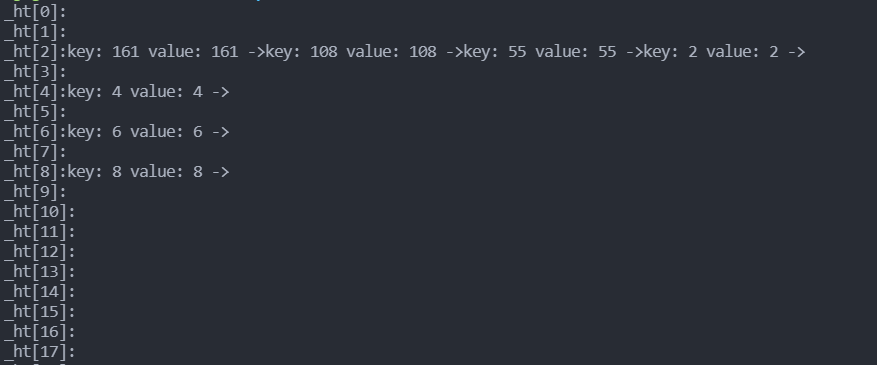4. 开散列与闭散列的比较
用链地址法处理哈希冲突，需要增加链接的指针，似乎增加了存储开销。事实上：由于开地址法必须保持大量的空闲空间来确保搜索效率，如二次探查法要求装载因子a<=0.7,而表项所占空间又比指针大的多，所以使用链地址法反而比开地址法节省存储空间
5. 存储其他类型
// 哈希函数可以采用处理余数法，key要化为整型才可以处理
// 仿函数
template<class T>
class DefHashF
{
public:
size_t operator()(const T& val)
{
return val;
}
};

// key为字符串类型，需要将其转化为整型
class Str2Int
{
public:
size_t operator()(const string& s)
{
// 将string类型转化为char*类型
const char* str = s.c_str();
unsigned int seed = 131;
unsigned int hash = 0;
while(*str)
{
hash = hash * seed + (*str++);
}
return (hash & 0x7FFFFFFF);
}
};
// 关键是s.c_str()函数

参考文章
unorder（哈希-海量数据处理)模拟实现hashtable
展开全文c++
• 哈希表：将无限的key转化为整型(通过散列函数)，存储在有限的数组空间中。最重要的是散列函数的构造和哈希冲突的解决。


目录标题
哈希表(散列表)一、引子二、定义三、散列函数（哈希函数) 的构造方法考虑因素1. 直接定址法2. 除留余数法3. 数字分析法4. 折叠法5. 平方取中法6. 字符关键词的散列函数构造
四、冲突处理方法思路开放定址法1. 线性探测法2. 平方探测法3. 双散列探测法4. 再散列5. 删除操作
分离链接法
五、散列表查找性能分析1. 成功与不成功平均查找长度2. 影响因素3. 线性探测法的查找性能4. 平方探测法和双散列探测法的查找性能5. 期望探测次数与装填因子α的关系6. 分离链接法的查找性能7. 分析
参考文章

哈希表(散列表)
一、引子
编译处理时，涉及变量及属性(如：变量类型)的管理：
插入：新变量定义查找：变量的引用
变量管理时，需要动态查找，但是利用查找树(搜索树)进行管理，比起整数的比较，两个变量名(字符串) 比较的效率不高，如果可以将字符串通过某个函数转化为整数来比较，效率就会提高
已知的几种查找方法：
查找方法时间复杂度顺序查找O(N）二分查找(静态查找)O(log2N）二叉搜索树O(h) h为二叉查找树的高度平衡二叉树O(log2 N)
散列查找的时间复杂度几乎是常量：O（1），即查找时间与问题规模无关 如果没有溢出(冲突)，T(查询) = T(插入) = T(删除) = O(1）
二、定义
散列表(Hash table,也叫哈希表)，是根据 键值（key）来直接进行访问的数据结构。 通过把关键码值映射到表中一个位置来访问记录，以加快查找的速度。这个映射函数叫做散列函数，存放记录的数组叫做散列表  哈希表就是将key值通过一个固定的算法函数(即散列函数)转化为一个整型数字，然后就将该数字对数组长度进行取余，取余结果就当做数组的下标，将value存储在以该数字为下标的数组空间里 查找数据时也是利用哈希函数  散列(Hashing)的基本思想是： （1）以关键字key为自变量，通过一个确定的函数h（散列函数)，计算出对应的函数值h(key)，作为数据对象的存储地址。 （2）可能不同的关键字会映射到同一个散列地址上，即h(keyi) = h(keyj)(但keyi != keyj )，称为 “冲突”  装填因子(Loading Factor): 设散列表空间大小为m，填入表中元素个数是n，则称α = n/m为散列表的装填因子
散列查找法的两项基本工作：
计算位置：构造散列函数确定关键词存储位置解决冲突：应用某种策略解决多个关键词位置相同的问题
三、散列函数（哈希函数) 的构造方法
考虑因素
一个“好”的散列函数一般应考虑下列两个因素： 1.计算简单，以便提高转换速度； 2. 关键词对应的地址空间要分步均匀，以尽量减少冲突
1. 直接定址法

直接确定函数

取关键词的某个线性函数值为散列地址，即 h(key) = a x key + b (a,b 为常数)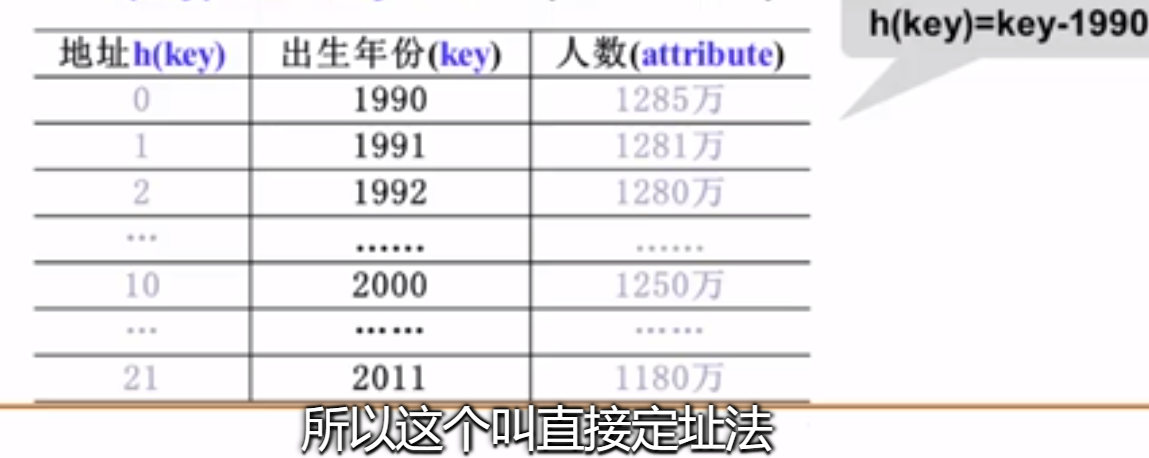2. 除留余数法
散列函数为：h(key) = key mod p 例：h(key) = key % 17 素数就是不能被1以上整除的数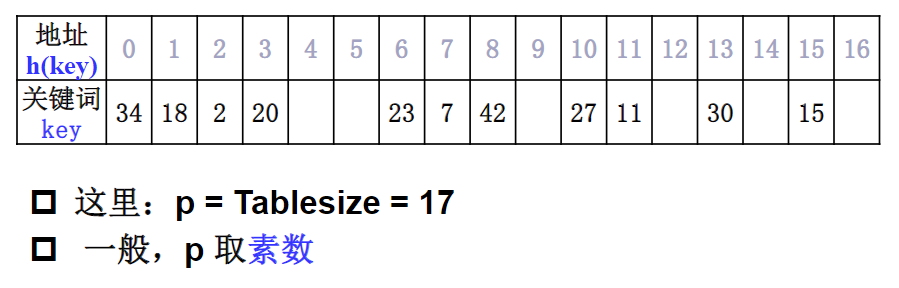3. 数字分析法
分析出对象的关键字在每一位上的表现，我们把那些能够随机变化的这些位取出来，组成我们的地址，达到映射均匀的这样一个目的。 例子如下：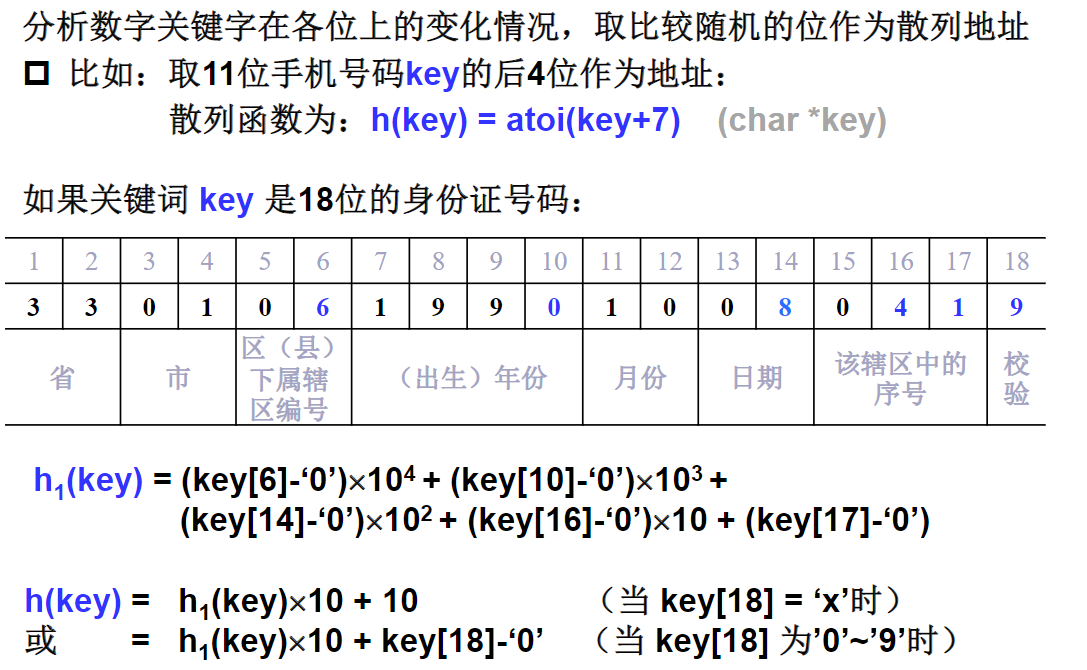4. 折叠法
把关键词分割成位数相同的几个部分，然后叠加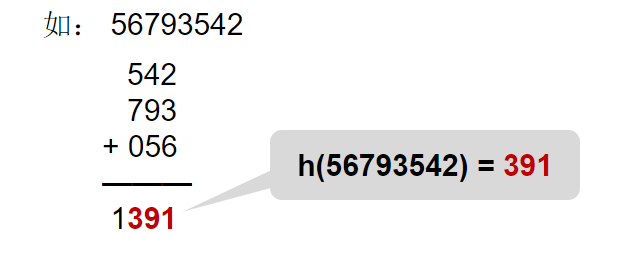5. 平方取中法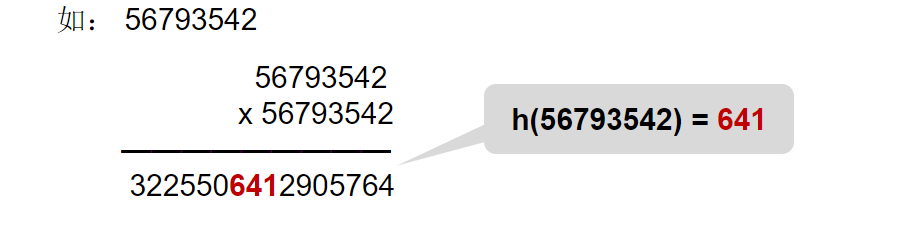6. 字符关键词的散列函数构造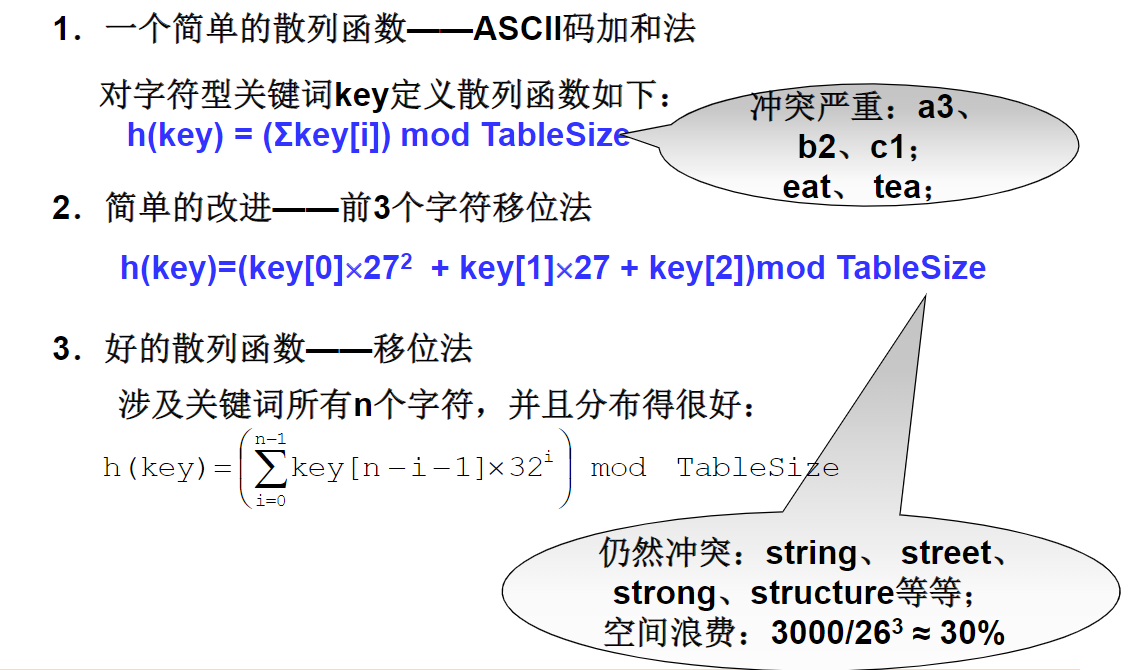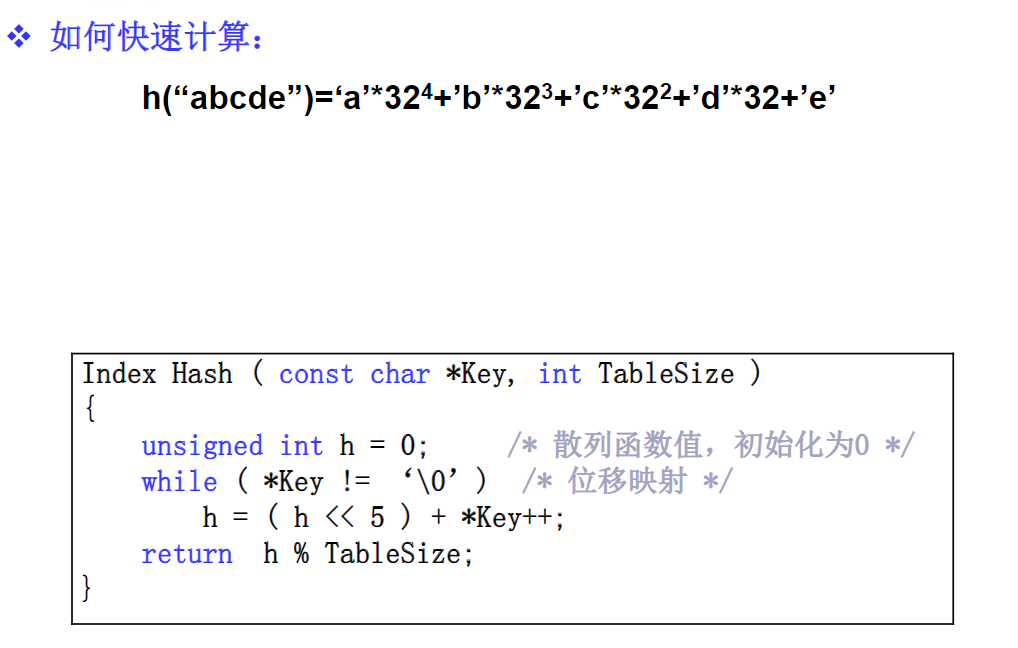四、冲突处理方法
思路
冲突就是不同的key通过哈希函数得到了相同的值，但要存储的空间已经被占据了，需要解决这个问题。
常用处理冲突的思路：
换个位置：开放地址法同一位置的冲突对象组织在一起：链地址法
开放定址法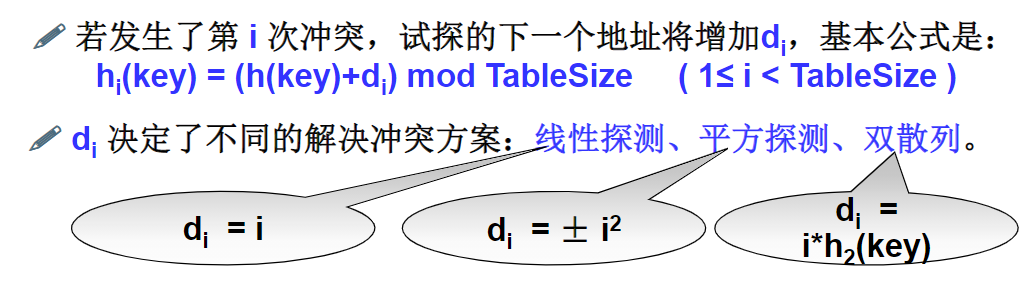1. 线性探测法
线性探测法：以增量序列1,2，…,（TableSize-1)循环试探下一个存储地址。
2. 平方探测法
平方探测法：以增量序列1^2, (-1)^2, 2^2, (-2)^2, …, q^2, (-q)^2 且q<=[TableSize/2] 循环试探下一个存储地址

有定理显示：如果散列表长度TableSize是某个4k+3（k是正整数)形式的素数时，平方探测法就可以探查到整个散列表空间

3. 双散列探测法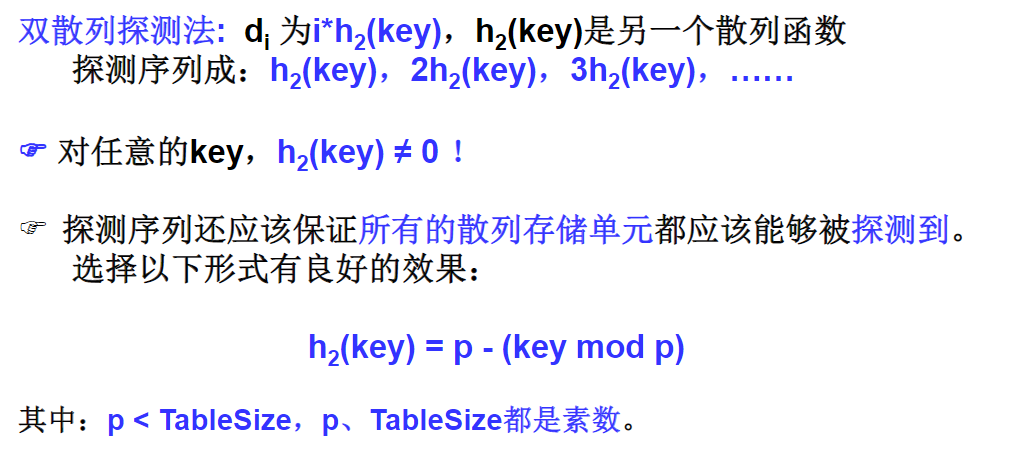4. 再散列
当散列表元素太多(即装填因子α太大)时，查找效率会下降； 》实用最大装填因子一般取0.5 <= α <= 0.85当装填因子过大时，解决的方法是加倍扩大散列表，这个过程叫 “再散列"
5. 删除操作
在开放地址散列表中，删除操作要很小心。通常只能"懒惰删除",即需要增加一个删除标记(Deleted)，而不是真正删除它。以便查找时不会”断链”。其空间可以在下次插入时重用。
分离链接法
分离链接法：将相应位置上冲突的所有关键词存储在同一个单链表中
五、散列表查找性能分析
1. 成功与不成功平均查找长度
成功平均查找长度(ASLs)不成功平均查找长度(ASLu)
例子如下：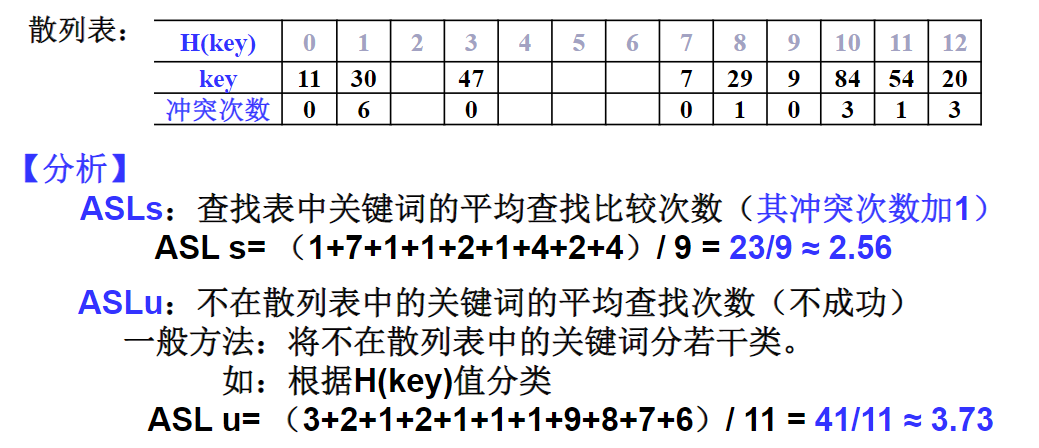成功平均查找长度就是每个关键词的冲突次数+1，然后全部相加取平均。 不成功平均查找长度就是 从开始位置查找，直到地址是空的位置，这个过程的查找次数就是不成功查找长度
2. 影响因素
影响产生冲突多少有以下三个因素： （1）散列函数是否均匀 （2） 处理冲突的方法 （3）散列表的装填因子α
3. 线性探测法的查找性能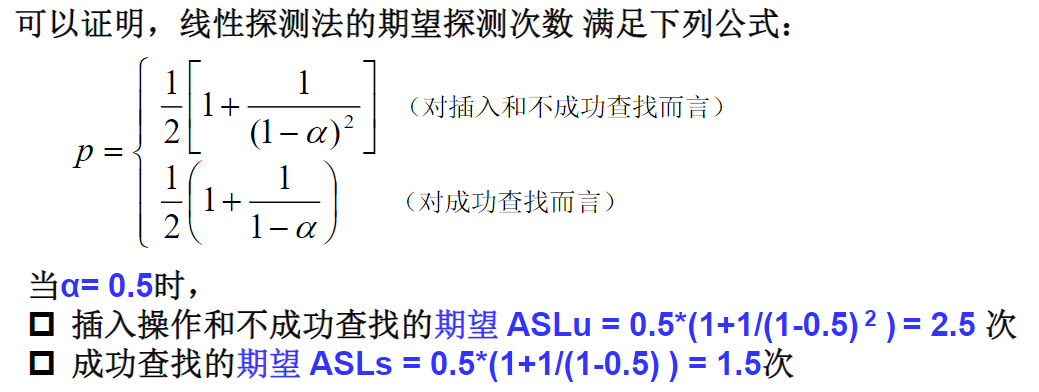4. 平方探测法和双散列探测法的查找性能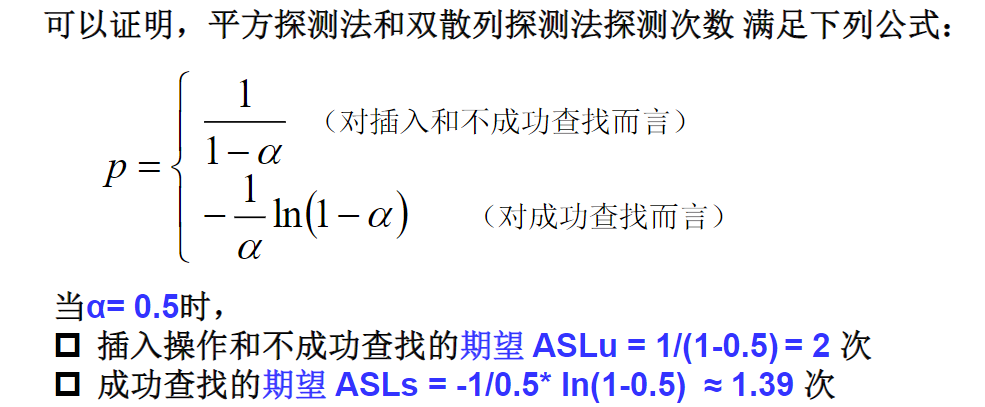5. 期望探测次数与装填因子α的关系
合理的最大装入因子α应该不超过0.85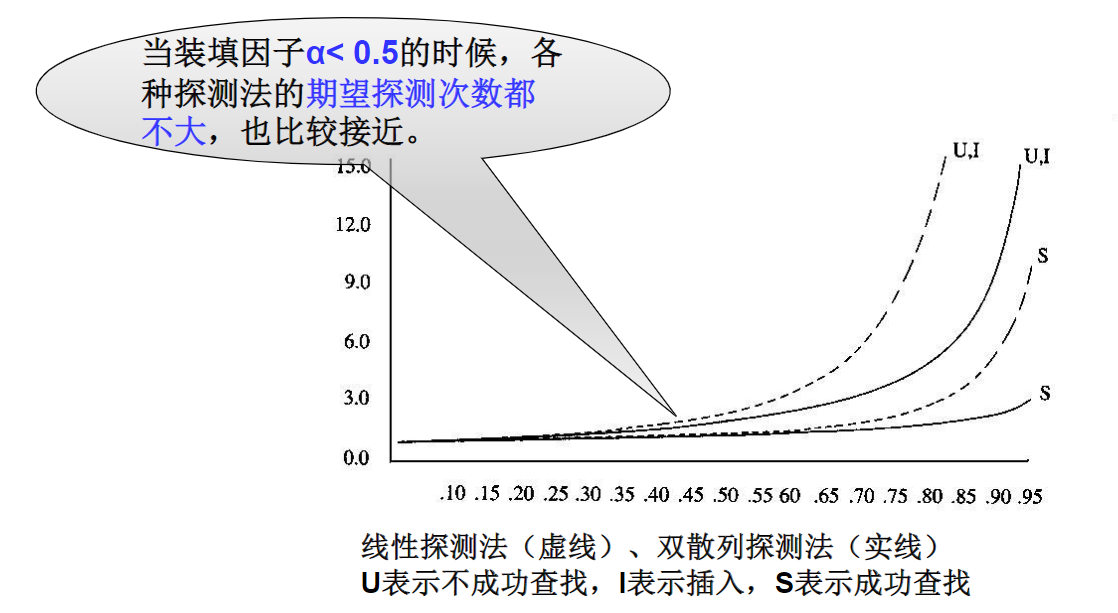6. 分离链接法的查找性能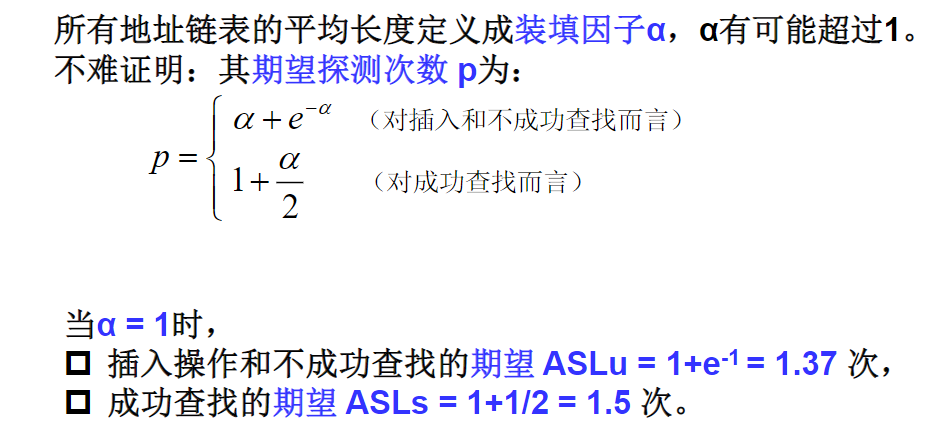7. 分析
开放地址法： 散列表是一个数组，存储效率高，随机查找 散列表有"聚集"现象分离链接法： 散列表是顺序存储和链式存储的结合，链表部分的存储效率和查找效率都比较低 关键字删除不需要"懒惰删除“法，从而没有存储"垃圾" 太小的α可能导致空间浪费，大的α又将付出更多的时间代价。不均匀的链表长度导致时间效率的严重下降
参考文章
哈希表(散列表)原理详解数据结构_浙江大学_中国大学MOOC（慕课)
展开全文• 哈希桶 #include<iostream> #include<vector> using namespace std; //开散列 //hash表封装了一个链表指针数组，和一个size（存放的元素个数） //链表指针数组里面就是单链表的节点 //单链表节点 ...hash hashmap 链表 指针
• 什么是数据结构？  数据结构（Data Structure）是一门研究数据的组织和管理的学科。往往从外在表现为一组数据的集合或者容器。 概念解释 元素（Element）：被管理的原子数据，元素类型不限。 集合（Collection）：...队列 二叉树
•算法 c语言
• 数据结构中数据元素和数据项的区别为：性质不同、组成不同、单位级别不同。 一、性质不同 1、数据元素：数据元素是用一组属性描述定义、标识、表示和允许值的一个数据单元。在计算机程序中通常作为一个整体进行...
• 复杂数据结构的基础构建块 缺点 固定尺寸 插入/删除或重新排序值昂贵 排序效率低下 应用领域 基本电子表格 在复杂的结构中，例如哈希表 有关更深入的说明，请参阅有关数组的Edpresso文章！（地址：...
• 本片博客没有相关的代码，只是数据结构知识的总结，依照严蔚敏老师的数据结构教材和我的20篇博客做的一个总结。首先，我想根据教材的目录走一篇大体的框架。大家在网上可以看到很多的网课，其中的目录也只是几个大的...算法
• 将任意长度的二进制值串映射为固定长度的二进制值串，这个映射的规则就是哈希算法，而通过原始数据映射之后得到的二进制值串就是哈希值。 哈希算法的应用非常非常多，这里选了最常见的七个，分别是安全加密、唯一...
• 索引的本质就是一种排好序的数据结构。这个肯定都明白，自然而言就联想到字典中的目录 在详细讲解下面的索引数据前补充一句： 通过不断缩小想要获取数据的范围来筛选出最终想要的结果，同时把随机的事件变成顺序事件...数据库 链表 二叉树
• 数据结构_栈 数据结构_队列 数据结构_数组 查询块:因为通过地址值查找内容 增删慢:因为增删操作会频繁的创建新的数组 数据结构_链表 数据结构_红黑树 List接口 Collection接口子接口List接口 List接口的特点: ...列表 链表 java
• 1、什么是哈希算法？  哈希算法的定义和原理：将任意长度的二进制...从哈希值不能反向推导出原始数据（所以哈希算法也叫单向哈希算法） 对输入的数据比较敏感，原始数据即使修改一个字节，最后得到的哈希值也大不...
• 我的数据结构课程的第三个项目基于树，堆等数据结构。 这是土耳其语中的问题定义： 项目3搜索树，桩和哈希表：自行车租赁系统每个学生都将做好准备，将程序打包并加载到系统中，并将它们带入报告中，并将所请求的...
• 文章主要分为两大部分，第一部分介绍了Redis对象的各种底层数据结构，第二部分总结了redis对象与各种底层数据结构的关系。 1 Redis对象底层数据结构 1.1 SDS(简单动态字符串) Redis没有直接使用C语言传统的字符...Redis
• 这里写目录标题线性结构和 非线性结构稀疏数组SparseArray需求介绍实例代码实现代码执行结果队列介绍数组模拟队列思路代码实现问题数组模拟环形队列环形队列代码实现 线性结构和 非线性结构 稀疏数组SparseArray ...算法 java
• ## RocketMQ

万次阅读 多人点赞 2019-07-31 19:17:34
在 RocketMQ 中，所有消息队列都是持丽化，长度无限的数据结构，所谓长度无限是挃队列中的每个存储单元都是定长，访问其中的存储单元使用 Offset 来访问，offset 为 java long 类型，64 位，理论上在 100年内不会...RocketMQ
• 标题已经说过，本文是对数据结构中的指针相关问题的解析。说了这么多最终必然还要回归数据结构（本文不详细展开数组中指针的使用）。 这里有个建议，大家在日常学习的时候，最好还是尽可能去搜索一些本源的知识，...算法 指针
• 关于哈希定义哈希冲突新的改变功能快捷键合理的创建标题，有...简单来说，哈希表就是根据关键码去寻找值的数据结构。类似于字典的结构：要寻找“按”，首先在字典索引中寻找拼音“an”，找到所在页数，即根据key，找到
• 今天整理出一些算法相关学习资源，包括书籍、算法刷题网站、项目资源、... 对于每一章的知识，先阅读标题，弄懂大概讲的是什么主题，再去快速看一遍，不懂也没有关系，但是一定要在不懂的地方做个记号，什么记号无所...
• 输入数据第一行为两个正整数分别为：哈希表表长m（m<100）和除数p（p<=m）。后面每一行是一个整数关键字，以-1作为输入的结束。 输出： 若输入的关键字在哈希表中已存在，则输出该关键字在哈希表中的位置，...算法
• 数据结构1800刷题????错题集 序号标题为解答，引用为题目和答案 多型就是数据元素的类型不确定 以下哪个数据结构不是多型数据类型（ D）【中山大学1999 一、3（1 分）】 A．栈B．广义表C．有向图D．字符串 ...算法
• ## Redis面试题集

千次阅读 多人点赞 2019-09-16 10:19:31
合理的创建标题，有助于目录的生成如何改变文本的样式插入链接与图片如何插入一段漂亮的代码片生成一个适合你的列表创建一个表格设定内容居中、居左、居右SmartyPants创建一个自定义列表如何创建一个注脚注释也是必...
• 这里写自定义目录标题欢迎使用Markdown编辑器新的改变功能快捷键合理的创建标题，有助于目录的生成如何改变文本的样式插入链接与图片如何插入一段漂亮的代码片生成一个适合你的列表创建一个表格设定内容居中、居左、...算法
• 数据结构与算法1课后题整理第一章什么是数据结构、算法结构与设计功能快捷键合理的创建标题，有助于目录的生成如何改变文本的样式插入链接与图片如何插入一段漂亮的代码片生成一个适合你的列表创建一个表格设定内容...算法
• 逻辑结构和物理结构是数据结构中重要的两个概念。 所谓逻辑结构，简单来说就是理解上的两个数据元素的关系，它很直观。学术点说就是数据对象中两个数据元素之间的相互关系，一般可以用一种偏序表示方法进行表示。...
• 这里写目录标题简单动态字符串（SDS）优点:链表特点字典特点哈希冲突的解决方式跳跃表特点压缩列表特点 简单动态字符串（SDS） struct sdshdr { // 记录buf数组中已使用字节的数量 int len; // 记录buf数组中未...redis 链表
• Python3 (入门2) 数据结构 本文由 Luzhuo 编写,转发请保留该信息. 原文: http://blog.csdn.net/rozol/article/details/68938984 以下代码以Python3.6.1为例 Less is more! #!/usr/bin/env python #...python
• 类可以认为是对结构的扩充，它和C中的结构最大的不同是：类中不但可以包括数据，还包括处理这些数据的函数。类是对数据和处理数据的方法（函数）的封装。类是对某一类具有相同特性和行为的事物的描述。例如，定义一...c#...

# 数据结构哈希表题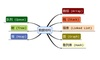数据结构 订阅